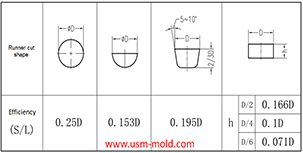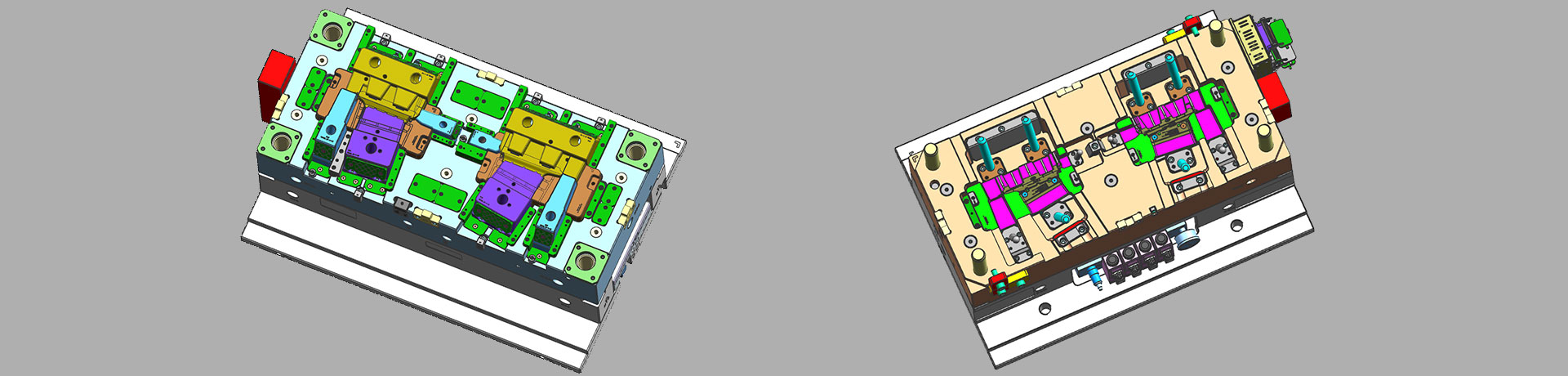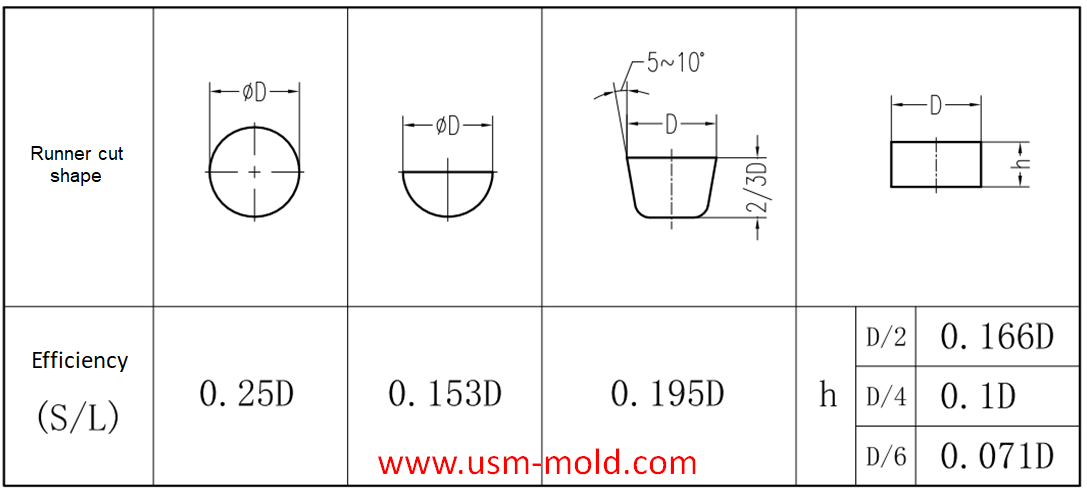﻿ Hydraulic diameter conversion of runners in plastic mold gating system design - UNIQUE SOLUTIONS MOLD LIMITED0769-8539-0209 / +86-15158220988
En# Hydraulic diameter conversion of runners in plastic mold gating system design

Hydraulic diameter refers to 4 times the ratio of the flow cross-sectional area to the perimeter, as the wall shear stress of non-circular pipes is not even distributed along the surrounding walls, only the average value of the surrounding walls can be calculated.

The main cross-sectional shapes of the shunt: round, trapezoidal and rectangular, in order to reduce the pressure heat loss in the runner, the cross-sectional area of the runner should be maximized, and the internal surface area for heat dissipation should be the smallest.

The efficiency of the runner is expressed by the ratio of the cross-sectional area S of the runner to its cross-sectional circumference L.

Splitter efficiency (hydraulic radius) n=cross-sectional area/cross-sectional circumference=S/L

Hydraulic diameter=4n=4S/L

From the above information, we can see the flow efficiency of different cross-section runners, round and trapezoidal cross-sections are the preferred runners. The size of the runner is usually selected based on the diameter of the circular runner (equal to the hydraulic diameter); when other cross-section flow channels are used, the hydraulic diameter must be converted so that the flow efficiency can meet the calculation requirements (see the example below) ).

Example: It is calculated that a product needs a 8m hydraulic diameter runner, and a semicircular runner is now used; what size of semicircle is more reasonable?

Hydraulic diameter=4n=4S/L=(4*(πr²)/2)/(π*r+2r)=8